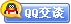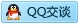##用户名 Email 自动登录 找回密码 密码 会员注册
 VIP会员，3年作业免费下 ！ 奥鹏作业，奥鹏毕业论文检测 新手作业下载教程，充值问题 没有找到答案，请在此处留言！ 2020年07月最新全国统考资料 投诉建议，加盟合作！奥鹏课程积分软件(ver:3.1)

# 浙大12春《微积分(2)》在线作业发表于 2012-3-14 14:45:33 | 显示全部楼层 |阅读模式谋学网: www.mouxue.com 主要提供奥鹏作业答案，奥鹏在线作业答案，奥鹏离线作业答案以及奥鹏毕业论文，致力打造中国最专业远程教育辅导社区。 一、单选题（共 20 道试题，共 40 分。）V 1.  若函数f(x,y)在(x0,y0)的某邻域内连续，则函数f(x0,y) ( ) A. 在y0点连续 B. 在y0点可导 C. 在y0点可微 D. 在y0点取得极值       满分：2  分 2.  已知函数y= 2xsin3x-5e^(2x), 则x=0时的导数y'=（ ） A. 0 B. 10 C. -10 D. 1       满分：2  分 3.  微分方程ydx+xdy=0的通解是( ) A. xy=C B. xy=0 C. x+y=C D. x-y=0       满分：2  分 4.  已知y= 4x^3-5x^2+3x-2, 则x=0时的二阶导数y"=（ ） A. 0 B. 10 C. -10 D. 1       满分：2  分 5.  已知z= 3cos(cos(xy)),则x=0,y=0时的全微分 dz =（ ） A. dx B. dy C. 0 D. dx+dy       满分：2  分 6.  微分方程y'=2x+sinx的一个特解是（ ） A. y=x^2+cosx B. y=x^2-cosx C. y=x+cosx D. y=x-cosx       满分：2  分 7.  设f(x)是可导函数，则（） A. ∫f(x)dx=f'(x)+C B. ∫[f'(x)+C]dx=f(x) C. [∫f(x)dx]'=f(x) D. [∫f(x)dx]'=f(x)+C       满分：2  分 8.  微分方程dy/dx=1+y/x+y^2/x^2 是（ ） A. 一阶齐次方程，也是伯努利方程 B. 一阶齐次方程，不是伯努利方程 C. 不是一阶齐次方程，是伯努利方程 D. 既不是一阶齐次方程，也不是伯努利方程       满分：2  分 9.  已知函数y= 2cos3x-5e^(2x), 则x=0时的微分dy=（ ） A. 10 B. 10dx C. -10 D. -10dx       满分：2  分 10.  已知z= 3sin(sin(xy)),则x=0,y=0时的全微分 dz =（ ） A. dx B. dy C. dx+dy D. 0       满分：2  分 11.  函数y=|x-1|+2的极小值点是( ) A. 0 B. 1 C. 2 D. 3       满分：2  分 12.  设函数f(x-2)=x^2+1，则f(x+1)=( ) A. x^2+2x+2 B. x^2-2x+2 C. x^2+6x+10 D. x^2-6x+10       满分：2  分 13.  设函数f(x)是在[-m,m]上的连续偶函数，且f(x)≠0,F(x)=∫f(t)dt,{积分区间是a->x}则F(x)（ ） A. 必是奇函数 B. 必是偶函数 C. 不可能是奇函数 D. 不可能是偶函数       满分：2  分 14.  由曲线y=cosx (0=

### 本帖子中包含更多资源

x
 本版积分规则 回帖并转播 回帖后跳转到最后一页客服一客服二客服三客服四微信客服扫一扫